# Segment, ShapeContour and Shape

OPENRNDR offers a lot of tools for creating and drawing two dimensional shapes.

## Segment

The basic element for constructing shapes is the `Segment`: a Bézier curve with a start point, an end point and zero, one or two control points.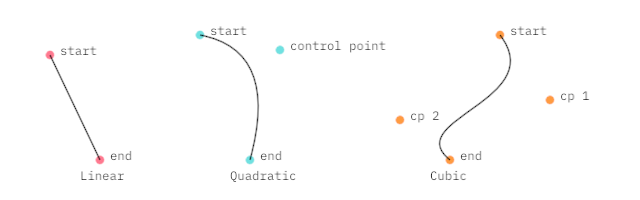### Constructing segments

``````// Linear Segment: start point, end point
val seg1 = Segment(Vector2(50.0, 55.0), Vector2(100.0, 160.0))

// Quadratic Segment: start point, control point, end point
val seg2 = Segment(Vector2(200.0, 35.0), Vector2(280.0, 50.0), Vector2(250.0, 160.0))

// Cubic Segment: start point, control point, control point, end point
val seg3 = Segment(Vector2(500.0, 35.0), Vector2(550.0, 100.0), Vector2(400.0, 120.0), Vector2(450.0, 160.0))
``````

### Drawing segments

``````// Draw one segment
drawer.segment(seg3)

// Draw multiple segments
drawer.segments(listOf(seg1, seg2, seg3))
``````

Note that `Segment`, like `Circle`, `Rectangle` and other geometric entities in OPENRNDR, are mathematical representations which can be rendered to the screen, but this is not necessary. A reason to create such geometries without displaying them is to serve as building blocks for constructing more complex designs. We can achieve this by querying curve properties.

### Segment properties

The `Segment` class provides multiple methods to query its properties. In the following examples, the `ut` argument is a normalized value that indicates a position in the segment between 0.0 (at the start) and 1.0 (at the end).

``````// Get a point on the curve near the start.
val pos = seg.position(ut = 0.1)

// Obtain the normal vector near the end.
// This is a vector of length 1.0 perpendicular to the curve.
val normal = seg.normal(ut = 0.9)

// Get the bounding box of the curve as a Rectangle instance.
val rect = seg.bounds

// Obtain the length of the curve.
val length = seg.length

// Get the point on the curve which is nearest to a given point.
val nearest = seg.nearest(Vector2(50.0, 50.0)).position

// Get 20 equally spaced curve points
val points = seg.equidistantPositions(20)
``````

The list of available methods can be found at the API website or in the source code.

### Modifying segments

Several methods return a new Segment based on the original one.

``````// Split a segment at the center returning two segments
val segments = seg.split(0.5)

// Get the center part of a segment
val subSegment = seg.sub(0.25, 0.75)

// Get the segment reversed (the start becomes the end)
val revSegment = seg.reverse

// Get the segment offset by the given distance
val offsetSegment = seg.offset(5.0)
``````

## ShapeContour

A `ShapeContour` is a collection of `Segment` instances in which each segment ends where the next one starts. A ShapeContour can be closed like the letter O or open like the letter S. It can be used to describe simple shapes like a square, or more complex ones.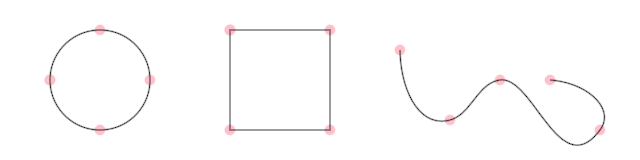Three ShapeContours with 4 segments each. The one on the right is open.

### Constructing a ShapeContour using the ContourBuilder

The `ContourBuilder` class offers a simple way of producing complex two dimensional shapes. It employs a vocabulary that is familiar to those who have used SVG.

• `moveTo(position)` move the cursor to the given position
• `lineTo(position)` insert a line contour starting from the cursor, ending at the given position
• `moveOrLineTo(position)` move the cursor if no cursor was previously set or draw a line
• `curveTo(control, position)` insert a quadratic bezier curve starting from the cursor, ending at position
• `curveTo(controlA, controlB, position)` insert a cubic bezier curve starting from the cursor, ending at position
• `continueTo(position)` inside a quadratic bezier curve starting from the cursor and reflecting the tangent of the last control
• `continueTo(controlB, position)` insert a cubic spline
• `arcTo(radiusX, radiusY, largeAngle, sweepFlag, position)`
• `close()` close the contour
• `cursor` a `Vector2` instance representing the current position
• `anchor` a `Vector2` instance representing the current anchor

Let’s create a simple `Contour` and draw it. The following program shows how to use the contour builder to create a triangular contour.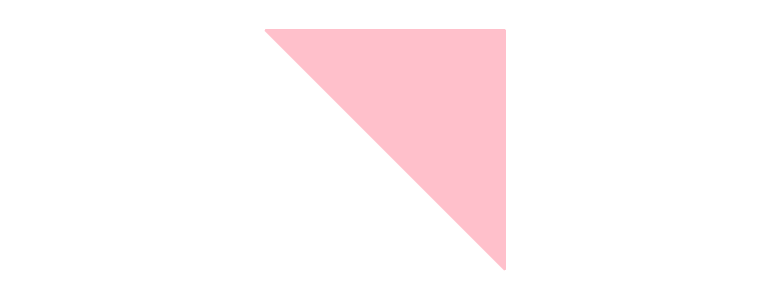``````fun main() = application {
program {
extend {
val c = contour {
moveTo(Vector2(width / 2.0 - 120.0, height / 2.0 - 120.00))
// -- here `cursor` points to the end point of the previous command
lineTo(cursor + Vector2(240.0, 0.0))
lineTo(cursor + Vector2(0.0, 240.0))
lineTo(anchor)
close()
}
drawer.clear(ColorRGBa.WHITE)
drawer.fill = ColorRGBa.PINK
drawer.stroke = null
drawer.contour(c)
}
}
}
``````

### Constructing a ShapeContour from points

We can use `.fromPoints()` to connect points with straight segments.

The `hobbyCurve` method, found in `orx-shapes`, can be used to create smooth curves.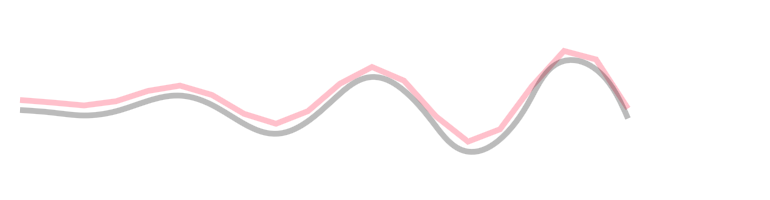``````fun main() = application {
program {
val points = List(20) {
Vector2(20.0 + it * 32.0, 100.0 + sin(it * 1.0) * it * 3)
}
val wavyContour = ShapeContour.fromPoints(points, closed = false)
val smoothContour = hobbyCurve(points, closed = false)

extend {
drawer.clear(ColorRGBa.WHITE)
drawer.fill = null
drawer.strokeWeight = 5.0
drawer.stroke = ColorRGBa.PINK
drawer.contour(wavyContour)

drawer.translate(0.0, 10.0) // displace 10px down
drawer.stroke = ColorRGBa.BLACK.opacify(0.5)
drawer.contour(smoothContour)
}
}
}
``````

### Constructing a ShapeContour from segments

Notice how each segment starts where the last one ends.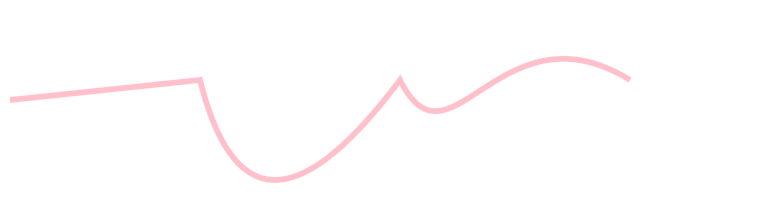``````fun main() = application {
program {
val segments = listOf(Segment(Vector2(10.0, 100.0), Vector2(200.0, 80.0)), // Linear Bézier Segment
Segment(Vector2(200.0, 80.0), Vector2(250.0, 280.0), Vector2(400.0, 80.0)), // Quadratic Bézier segment
Segment(Vector2(400.0, 80.0), Vector2(450.0, 180.0), Vector2(500.0, 0.0), Vector2(630.0, 80.0))) // Cubic Bézier segment
val horizontalContour = ShapeContour.fromSegments(segments, closed = false)

extend {
drawer.clear(ColorRGBa.WHITE)
drawer.strokeWeight = 5.0
drawer.stroke = ColorRGBa.PINK
drawer.contour(horizontalContour)
}
}
}
``````

### Constructing a ShapeContour from a primitive

Primitives like `Rectangle`, `Circle` and `LineSegment` can be easily converted into a `ShapeContour`.

``````val c1 = Circle(200.0, 200.0, 50.0).contour
``````

### Even more ways to construct a ShapeContour

Take a look at `orx-turtle` and `orx-shapes` for other ways to create contours, including regular polygons, rounded rectangles and more.

### Drawing a ShapeContour

``````// Draw one contour
drawer.contour(contour1)

// Draw multiple contours
drawer.contours(listOf(contour1, contour2, contour3))
``````

Note that if the contour is closed, the current fill color is used.

### ShapeContour properties

The `ShapeContour` provides methods to query its properties similar to the ones found in `Segment`.

``````// Get a point on the contour near the start.
val pos = contour.position(ut = 0.1)

// Obtain the normal vector near the end.
// This is a vector of length 1.0 perpendicular to the curve.
val normal = contour.normal(ut = 0.9)

// Get the bounding box of the curve as a Rectangle instance.
val rect = contour.bounds

// Obtain the length of the curve.
val length = contour.length

// Get the point on the curve which is nearest to a given point.
val nearest = contour.nearest(Vector2(50.0, 50.0)).position

// Get 20 equally spaced curve points
val points = contour.equidistantPositions(20)
``````

An example of using `.position()` and `.equidistantPositions()`:

``````fun main() = application {
program {
extend {
drawer.clear(ColorRGBa.WHITE)
drawer.stroke = null
drawer.fill = ColorRGBa.PINK

val point = Circle(185.0, height / 2.0, 90.0).contour.position((seconds * 0.1) % 1.0)
drawer.circle(point, 10.0)

val points0 = Circle(385.0, height / 2.0, 90.0).contour.equidistantPositions(20)
drawer.circles(points0, 10.0)

val points1 = Circle(585.0, height / 2.0, 90.0).contour.equidistantPositions((cos(seconds) * 10.0 + 30.0).toInt())
drawer.circles(points1, 10.0)
}
}
}
``````

The list of available methods can be found at the API website or in the source code.

### Modifying a ShapeContour

#### sub()

A contour can be cut into a shorter contour using `ShapeContour.sub()`.

``````fun main() = application {
program {
extend {
drawer.clear(ColorRGBa.WHITE)
drawer.fill = null
drawer.stroke = ColorRGBa.PINK
drawer.strokeWeight = 4.0

val sub0 = Circle(185.0, height / 2.0, 100.0).contour.sub(0.0, 0.5 + 0.50 * sin(seconds))
drawer.contour(sub0)

val sub1 = Circle(385.0, height / 2.0, 100.0).contour.sub(seconds * 0.1, seconds * 0.1 + 0.1)
drawer.contour(sub1)

val sub2 = Circle(585.0, height / 2.0, 100.0).contour.sub(-seconds * 0.05, seconds * 0.05 + 0.1)
drawer.contour(sub2)
}
}
}
``````

#### offset()

The function `ShapeContour.offset` can be used to create an offset version of a contour.

``````fun main() = application {
program {
// -- create a contour from Rectangle object
val c = Rectangle(100.0, 100.0, width - 200.0, height - 200.0).contour.reversed

extend {
drawer.fill = null
drawer.stroke = ColorRGBa.PINK
drawer.contour(c)
for (i in 1 until 10) {
val o = c.offset(cos(seconds + 0.5) * i * 10.0, SegmentJoin.BEVEL)
drawer.contour(o)
}
}
}
}
``````

`ShapeContour.offset` can also be used to offset curved contours. The following demonstration shows a single cubic bezier offset at multiple distances.

``````fun main() = application {
program {
val c = contour {
moveTo(width * (1.0 / 2.0), height * (1.0 / 5.0))
curveTo(width * (1.0 / 4.0), height * (2.0 / 5.0), width * (3.0 / 4.0), height * (3.0 / 5.0), width * (2.0 / 4.0), height * (4.0 / 5.0))
}
extend {
drawer.stroke = ColorRGBa.PINK
drawer.strokeWeight = 2.0
drawer.lineJoin = LineJoin.ROUND
drawer.contour(c)
for (i in -8..8) {
val o = c.offset(i * 10.0 * cos(seconds + 0.5))
drawer.contour(o)
}
}
}
}
``````

#### reversed, close(), transform(), …

For more properties and methods explore the API website or the source code.

## Shape

OPENRNDR uses `Shape` to represent planar shapes. We can think of a `Shape` as a group of `ShapeContour` instances, where each `ShapeContour` is a sequence of one or more Bézier `Segment`.

### Constructing a Shape using the shape builder

Let’s create a `Shape` using the shape builder. The shape is created using two contours, one for the outline of the shape, and one for the hole in the shape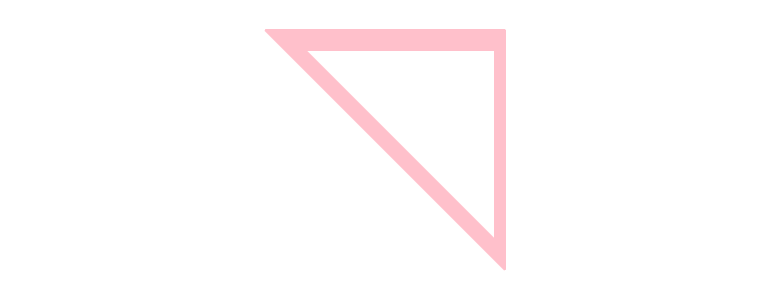``````fun main() = application {
program {
extend {
val s = shape {
contour {
moveTo(Vector2(width / 2.0 - 120.0, height / 2.0 - 120.00))
lineTo(cursor + Vector2(240.0, 0.0))
lineTo(cursor + Vector2(0.0, 240.0))
lineTo(anchor)
close()
}
contour {
moveTo(Vector2(width / 2.0 - 80.0, height / 2.0 - 100.0))
lineTo(cursor + Vector2(190.0, 0.0))
lineTo(cursor + Vector2(0.0, 190.00))
lineTo(anchor)
close()
}
}
drawer.clear(ColorRGBa.WHITE)
drawer.fill = ColorRGBa.PINK
drawer.stroke = null
drawer.shape(s)
}
}
}
``````

### Constructing a Shape from a primitive

Primitives like `Rectangle`, `Circle`, `LineSegment` and `ShapeContour` can be easily converted into a `Shape`.

``````val s = Circle(200.0, 200.0, 50.0).shape
``````

## Shape Boolean-operations

Boolean-operations can be performed on shapes using the `compound {}` builder. There are three kinds of compounds: union, difference and intersection, all three of them are shown in the example below.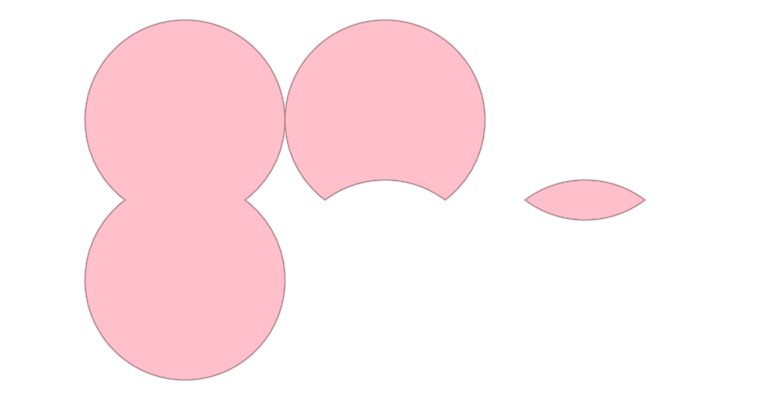``````fun main() = application {
program {
extend {
drawer.clear(ColorRGBa.WHITE)
drawer.fill = ColorRGBa.PINK

// -- shape union
val su = compound {
union {
shape(Circle(185.0, height / 2.0 - 80.0, 100.0).shape)
shape(Circle(185.0, height / 2.0 + 80.0, 100.0).shape)
}
}
drawer.shapes(su)

// -- shape difference
val sd = compound {
difference {
shape(Circle(385.0, height / 2.0 - 80.0, 100.0).shape)
shape(Circle(385.0, height / 2.0 + 80.0, 100.0).shape)
}
}
drawer.shapes(sd)

// -- shape intersection
val si = compound {
intersection {
shape(Circle(585.0, height / 2.0 - 80.0, 100.0).shape)
shape(Circle(585.0, height / 2.0 + 80.0, 100.0).shape)
}
}
drawer.shapes(si)
}
}
}
``````

The compound builder is actually a bit more clever than what the previous example demonstrated because it can actually work with an entire tree of compounds. Demonstrated below is the union of two intersections.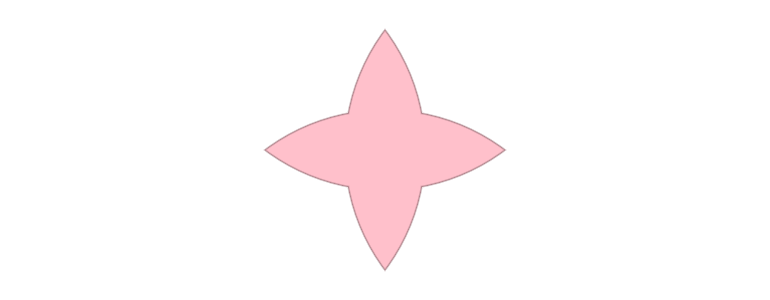``````fun main() = application {
program {
extend {
drawer.clear(ColorRGBa.WHITE)
drawer.fill = ColorRGBa.PINK
val cross = compound {
union {
intersection {
shape(Circle(width / 2.0 - 160.0, height / 2.0, 200.0).shape)
shape(Circle(width / 2.0 + 160.0, height / 2.0, 200.0).shape)
}
intersection {
shape(Circle(width / 2.0, height / 2.0 - 160.0, 200.0).shape)
shape(Circle(width / 2.0, height / 2.0 + 160.0, 200.0).shape)
}
}
}
drawer.shapes(cross)
}
}
}
``````

## Intersections

Extension methods are provided to find intersections between `Shape`, `ShapeContour` and `Segment` instances.

``````fun main() = application {
program {
extend {
// A rotation transformation to apply to the rectangle
val rotation = transform {
translate(width * 0.6, height * 0.5)
rotate(seconds * 18)
}

val circle = Circle(width * 0.4, height * 0.5, 80.0).contour
val rotatingRect = Rectangle.fromCenter(Vector2.ZERO, 150.0).contour.transform(rotation)

val intersections = circle.intersections(rotatingRect)

drawer.clear(ColorRGBa.WHITE)
drawer.strokeWeight = 2.0
drawer.stroke = ColorRGBa.PINK
drawer.fill = ColorRGBa.PINK.opacify(0.5)

drawer.contour(circle)
drawer.contour(rotatingRect)

// Draw intersections as small circles
drawer.fill = ColorRGBa.WHITE
drawer.stroke = ColorRGBa.BLACK.opacify(0.5)
drawer.circles(intersections.map {
it.position
}, 5.0)
}
}
}
``````